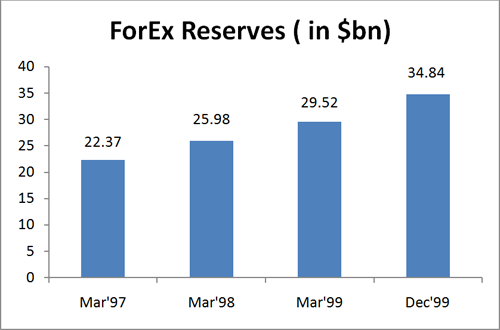# Data Interpretation: 5 C's of Success

Data Interpretation is a critical component of almost every competitive paper. Scoring in DI is not as tough as Mathematics, as the variety of questions asked is much lesser in DI.
In order to ensure speed and accuracy in the DI section, you must take care of the five C's of success given below.
1. Components: You must keep in mind that all information given in the graph/ table is important. You must read the graph/ table carefully; ensuring minor details, like units of quantities, are not overlooked. It is advised that you spend at least 10 - 15 seconds in the interpretation and understanding of table/ graph.
2. Co-relation: In many problem sets, there are two or more tables/ graphs given. Before you start solving questions, you should co-relate the graphs/ tables given and understand their relationship/ s and inter-dependencies.
3. Choices: Before solving any question you must always see the choices given. Spending 3-4 seconds to see the choices may reduce the time taken on each question by 40 - 50 seconds. By doing this, you may be able to eliminate one or more options. Keep in mind that even a fraction of a second saved during the exam is worth it!
4. Calculations: Try to make use of shortcuts and approximations by remembering important fractions to make calculation work fast and accurate. Intelligent guessing also helps in better time management in the DI section. The time spent on this section may be reduced up to 25 - 50% by intelligent and smart work.
5. Confidence: Most importantly, have confidence in yourself. For instance, if you are sure that the first, second and fourth options cannot be the answer; then straight away mark the third option as the answer. There is no need to further verify if the third option is the correct or not.
###### Data Interpretation - Bar Chart
What are Bar charts?
Bar charts are one of the easiest, graphically attractive and most commonly used methods of presenting different types of data. They are especially useful for representing data series. Questions based on such type of charts are common in competitive exams. Further, in Bar Charts, percent change and average based questions are generally seen in such exams.
DIRECTIONS for questions: Study the following graph and answer the questions given below.Example 1: The foreign exchange reserve in March 1998 increased by what percent as compared to the corresponding month last year?
A. 13.9%B. 21.7%C. 11.8%D. 16.1%
Solution: Foreign exchange reserve in March '98 = $25.98 bn. For corresponding period last year i.e. March '97, Foreign exchange reserve =$22.37 bn.
Increase in foreign exchange reserve = $3.61 bn. Percentage increase = 100 × 3.61/22.37 = 16.13%. Alternatively, Besides this, it can also be seen that 10% of the base i.e. 22.37 is 2.2 and 15% will be 3.3. Hence, the answer has to be slightly greater that 15%. Hence, answer will be fourth option. Example 2: The foreign exchange reserve in March 1997 was ---- % less than what was in March 1998. A. 16.2%B. 10.2%C. 13.9%D. 19.8% Solution: Foreign exchange reserve in March '98 =$25.98 bn
Foreign exchange reserve in March '97 = $22.37bn Decrease in foreign exchange reserve =$3.61bn
Percentage decrease = 100×3.16/25.98 = 13.9%
Alternatively,
Besides that, it can also be seen that 10% of the base i.e. 25.98 is approximately 2.6 and 15% will be 3.9. Hence, the answer has to be more than 10% and less than 15%. Hence, the third option is the answer.
Learning:
In both the examples, a quick glance on the Choices enables you to use approximations to solve the problem faster. However, had the options been very close to each other then approximations should be avoided.
Example 3: The average rate of increase per year of foreign exchange reserve in India from March 1997 to March 1999 was approximately
A. 10.5%B. 16%C. 26%D. 32%
Solution: Foreign exchange reserve in March' 97 = $22.37bn Foreign exchange reserve in March' 99 =$29.52bn
Increase in foreign exchange reserve = 7.15bn
Percentage increase in two years = 100× 7.15/22.37 = 32%
Average rate of increase per year = 32/2 = 16%
It can noted here that the increase of 7.15 is roughly one-third of 22 i.e. 33.33%. For every year it will become 33/2 = 16.5%. Hence, second option is the answer.
Learning:
As we see through the above question, all the Components of the bar chart are equally important.
Example 4: What was the average foreign exchange reserve for the three years i.e. March 1997, March 1998 and March 1999, approximately? ( in $mn) A. 26B. 22.18C. 2218D. 2600 Solution: From questions 1 and 2, the difference between March '97, '98 and '99 is almost same. Thus, the average would be the middle value i.e$25.98 billion = \$26 billion. However, since the answer is required in million, the answer is option D.
Learning:
For DI questions, it is essential to note the units of each component. In questions with similar options, always try and find the catch in the question, which in this case was the unit.
###### Key Learning:
In most of the cases, DI questions are NOT very calculation intensive. Keeping in mind these 5 C's you can easily solve any DI problem.
Practice different types of DI problems for improved accuracy and speed.